Click Question to Get Free Answers >

# Vector Algebra NCERT Solutions : Class 12 Maths

## NCERT Solutions for Class 12 Maths : Vector AlgebraFilter
Filters :
Classes
•
•
•
•
•
•
•
Chapters
•
•
•
•
•
•
•
•
•
•
• 3 More
Exercises
•
•
•
•
•
•
•

Doubtnut one of the best online education platform provides free NCERT Solutions of Maths for Class 12 Vector Algebra which are solved by our maths experts as per the NCERT (CBSE) guidelines. We provide the solutions as video solutions in which concepts are also explained along with answer which will help students while learning , preparing for exams and in doing homework. These Solutions will help to revise complete syllabus and score more marks in examinations.

Get here free, accurate and comprehensive NCERT Solutions for Class 12 Maths Vector Algebra which have been reviewed by our maths counsellors as per the latest edition following up the CBSE guidelines. We provide video solutions in which solutions to all the questions of NCERT Class 12 Maths textbook are explained in step by step and detailed way.

## The Topics of the Chapter Vector Algebra are :

PROPERTIES OF DOT PRODUCT

DOT PRODUCT

APPLICATIONS OF DOT (SCALAR) PRODUCT

VECTOR JONING TWO POINTS

POSITION VECTOR OF A POINT

VECTOR (OR CROSS) PRODUCT OF TWO VECTORS

TYPES OF VECTORS

DIRECTION COSINES AND DIRECTION RATIOS

EVOLUTION OF VECTOR CONCEPT

INTRODUCTION

SUBTRACTION OF VECTORS

MULTIPLICATION OF A VECTOR BY A SCALAR

SECTION FORMULA

COMPONENTS OF A VECTOR

PROPERTIES OF CROSS PRODUCT

SCALAR TRIPLE PRODUCT

MISCELLANEOUS

VECTOR TRIPLE PRODUT

LINEAR COMBINATION LINEAR INDEPENDENCE AND LINEAR DEPENDENCE

It contains these exercises along with solved examples. All the exercises are solved in the video. Select the exercise to view the solutions exercisewise of the Chapter Vector Algebra:

## NCERT Solutions Class 12 Maths Chapter Vector Algebra Exercises:

We have covered all the exercises and also Solved examples in the videos. Along with the practise exercise Students should also practise solved examples to clear the concepts of the Vector Algebra. If incase you have any doubt you can watch the solutions for the given questions.Watch the solutions for the given questions in which it is also explained in the video steps to solve the questions along with answers.

# NCERT Class 12 Solutions Chapter 10 Vector Algebra

Students, in this chapter, will get to know about the basic difference between the two major types of units that is vector and scalar, and what it actually means. It is very important to understand the chapter well in-depth, as it is a very significant chapter for further studies. If we talk about its importance and implication in different chapters and subjects, it is basically used in entire mechanics. Whatever students learn here in this chapter, they get to use it in different questions in mathematics as well as physics. Vector represents one of the most important mathematical systems, which is used to handle certain types of problems in geometry, mechanics and other branches of applied mathematics, physics and engineering. Here at Doubtnut, an e-learning platform, we are providing free and accurate NCERT Class 12 maths solutions.

The curriculum of CBSE board adheres to NCERT books; therefore, it makes the NCERT textbooks as the prime book to be studied for covering the entire topics of CBSE syllabus. The solved examples and theoretical explanations are presented in simple language in NCERT maths books. The students should go for NCERT textbook and practice the unsolved exercises regularly to attain a comprehensive understanding of the particular concepts. The NCERT book provides concise explanation of the concepts for a quick overview before beginning to solve problems. The NCERT book explains the concept in a lucid language that develop interests in students towards the particular chapter. The NCERT textbook has the updated question collection for the quick and easy revision of the students. The NCERT maths book provides a summary at the end of each chapter for a quick recap of the chapter.

Doubtnut is an e-learning platform that provides free NCERT solutions for the classes 6 to 12. Apart from the textbook solutions, Doubtnut also offers the learners with enough study material and mock tests for cracking competitive entrance examinations such as IIT-JEE, BITSAT. NCERT class 12 solutions chapter 10, Vectors Algebra, covers all the important concepts that come under this chapter. Each problem from the exercise is worked out in a step-by-step way and they are compiled by a team of experts.

The solutions are provided in video format and pdf format.  Hence, it provides the best and the easiest methods to solve the practice exercises. The intuitive video tutorials aid in effective learning and in-depth understanding of the concepts. Every example is provided with the use of graphics, images, and diagrams to make it clear to the students in an easy language. If students come across any doubts, all they have to do is just post pictures of their questions from the maths book on our website and the solution for the respective question will be provided.

## Topics & Subtopics of NCERT Class 12 Maths Chapter 10 – Vectors Algebra

### Scalar Quantity and Vector Quantity

If we talk about different physical quantities that are broadly divided as scalar and vector quantities. Those quantities which have only magnitude and are not related to any fixed direction in space is called scalar quantities or briefly scalar.

Examples of scalar quantities are mass, volume, density, work, temperature, etc. A scalar quantity is being represented by a real number along with a suitable unit. The second kind of quantities are those that have magnitude and direction, such quantities are called vectors. Displacement, velocity, acceleration, momentum, weight force, etc. are some of the examples of vector quantities.

Example:

Q-Classify the following measures as scalar and vectors?

1. 20 m north-west

2. 10 newton

3. 30 km/hr

4. 50 m/s towards the north

5. 10-19 Coulomb

A- 1. Directed distance -vector

2. Force – vector

3. Speed – scalar

4. Velocity – vector

5. Electric charge- scalar

### Representation of Vector

We represent the vector by a direct line segment.

For example, a= AB. Here, A is called the initial point and B is called the terminal point or tip.

A directed line segment with initial point A and terminal point B is denoted by AB or . Vectors are also denoted by small letters with an arrow above it or by small letters with an arrow above it or by small bold letter with an arrow above it or by small bold letters e.g. a, b, c, etc. or a, b, c (with arrow above these letters ), etc.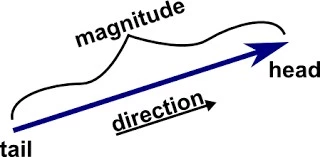Here, from head to tail magnitude is AB and it is expressed as (magnitude=|AB|) = AB (DISTANCE BETWEEN INITIAL AND TERMINAL POINTS)

REMARKS:

1. The magnitude of a vector is always a non- negative real number.

2. Every vector AB has the following three characteristics.

Length – The length of AB will be denoted by |AB| or AB.

Support- The line of unlimited length of which AB is a segment is called the support of the vector AB.

Sense – The sense of AB is from A to B and that of BA is from B to A. Thus, the sense of directed line segment from its initial point to its terminal point.

### TYPES OF VECTOR

1. Zero Or Null Vector: A vector whose magnitude is zero is called a null vector.
2. Unit Vector: A vector, whose modulus is unity, is called unity vector. Èƒ=a/|a|
3. Like And Unlike Vector: Vectors are said to be like when they have the same route of direction and unlike when they have opposite direction.
4. Collinear Or Parallel Vector: Vectors having the same of parallel supports are called collinear vectors.
5. Co-Initial Vectors: Vectors having the same initial points are called co-initial vectors.
6. Coplanar Vector: A system of vectors is said to be coplanar if they lie in the same plane or their supports are parallel to the same plane.
7. Coterminous Vector: Vectors having the same terminal points are called coterminous vectors.
8. Negative Of A Vector: The vector which has the same magnitude as the given vector a but opposite direction, is called the negative of a but opposite direction, is called the negative of a and is denoted by -a. Thus, if PQ=a, then QP= -a.
9. Reciprocal Of A Vector: The vector having the same direction as that of a given vector a but opposite direction, is called the negative of a and is denoted by -a.

REMARK:

A UNIT VECTOR IS SELF-RECIPROCAL.

POSITION VECTOR OF A POINT IN SPACE:

Consider a point whose location in space is specified with Cartesian Co-ordinates (e.g. P (x, y, z). Now consider the directed distance (a vector quantity) extending from the origin to this point.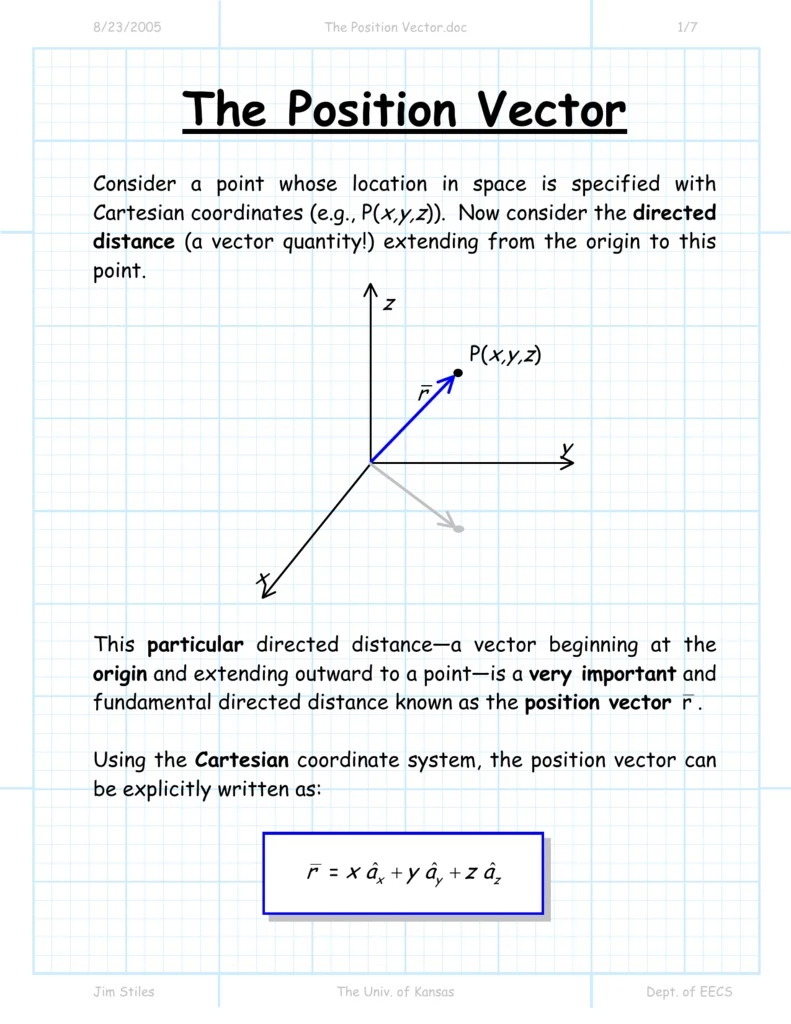This particular directed distance -a vector beginning at origin and extending toward to a point – is a very important and fundamental directed distance known as the position vector r -cap.

Using the Cartesian coordinate system the position vector can be explicitly written as:

r = xâx + yây + zÈƒz

DIRECTION COSINES: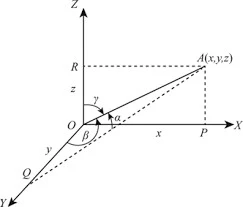The direction angles É‘, β, and É£ of a vector v= ai + bj + ck are defined as follows:

É‘ is the angle between v and the positive x-axis (0≤É‘≤π)

β is the angle between v and the position y-axis (0≤β≤π)

É£ is the angle between v and the positive z-axis (0≤É£≤π)

### ADDITION AND SUBTRACTION OF VECTORS, MULTIPLICATION OF VECTOR BY SCALAR, SECTION FORMULA

If two vectors are represented by two consecutive sides of a triangle, then their sum is represented by the third side of the triangle, but in the opposite direction. This is known as the triangle law of addition of vectors. Thus, if AB=a, BC=b, AC=c, then AB+BC=AC that is a+b=c.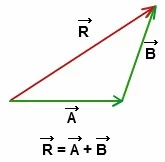If two vectors are represented by two adjacent sides of a parallelogram, then their sum is represented by the diagonal of the parallelogram whose initial point is the same as the initial point of the given vectors. This is known as the parallelogram law of vector addition.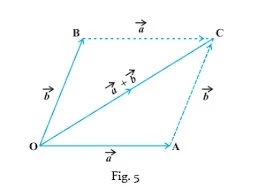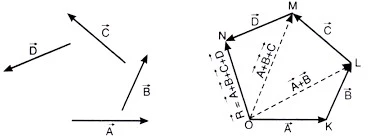AB+BC+CD+DE+EF=AF

• Commutativity: for any two vectors a b and c

a+b=b+a

• Associativity: for any two vectors a b and c

a+(b+c)=(a+b)+c

• Identity: zero vector is the identity for addition. For a vector a

0+a=a=a+0

• Additive Inverse: For every vector a its negative vector -a exists such that  a+(-a)=(-a)+a=0 that is (-a )is the additive inverse of the vector a.

### THEOREM OF NON-COPLANAR VECTORS:

Theorem 1:

If a, b and c are three non- zero, non-coplanar vectors and x, y, z are three scalars such that

xa + yb +zc = 0

Then x=y=z=0

Theorem 2:

If abc are non-coplanar vectors, then any vector r can be uniquely expressed as a linear combination xa + yb + zc; x, y and z being scalar.

### LINEAR INDEPENDENCE AND DEPENDENCE OF VECTORS

#### LINEARLY INDEPENDENCE VECTORS

It’s a set of non- zero vectors a1 a2..........an is said to be linearly independent, if

x1a1 + x2a2 +...........  +xnan = 0

x1 = x 2 = ........ = xn = 0

#### LINEARLY DEPENDENCE VECTORS

A set of vector a1 , a1 ....... an  is said to be linearly dependent , if exist scalar x1 ,x2,....... xn not all zero such that x1 a1 + x1 a2 + ........ + xn an = 0

### PRODUCT OF VECTOR

Let a and b be two non-zero, non-parallel vectors. Then the product of the vectors is a×b, in that order, is defined as a vector whose magnitude is

|a| |b| sin Ï´.

Where theta is the angle between a and b, whose direction is perpendicular to the plane of a and b in such a way that a, b and this direction constitutes a right-handed system.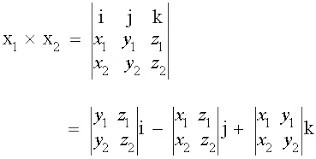CROSS PRODUCT OF A VECTOR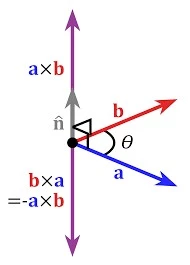#### DOT PRODUCT OF VECTOR

The other way to calculate the product of a vector is dot product for which if we take a dot product between a unit vector and itself, the angle is zero and cosÏ´ = 1. Given that the vectors are all of length one, the dot products are i.i = j.j = k.k=1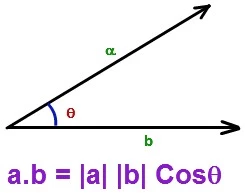Benefits in learning from Doubtnut

1. For further help, students can always visit our website for easy leaning and quick revisions of the chapter. Our website delivers free of cost solutions with conceptual explanation on the same.
2. We provide the entire solution of the NCERT textbook on our website for the benefit of the students and that helps in saving their precious time.
3. Video lectures are provided by subject matter experts, covering all the important topics and questions as per the examination point of view.
4. The language here is kept simple, no complicated terminology and no off the topic discussions. We provide an immersive learning experience at free of cost.